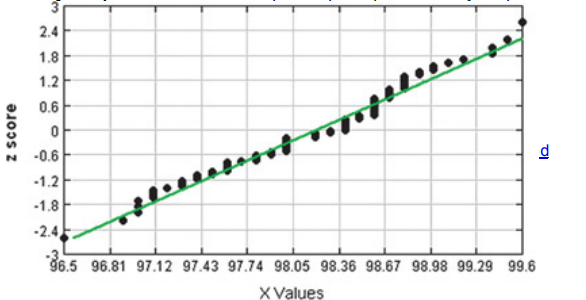×
Get Full Access to Elementary Statistics - 12 Edition - Chapter 6.6 - Problem 6bsc
Get Full Access to Elementary Statistics - 12 Edition - Chapter 6.6 - Problem 6bsc

×

Solved: Interpreting Normal Quantité Plots. In Exercises,ISBN: 9780321836960 18

Solution for problem 6BSC Chapter 6.6

Elementary Statistics | 12th Edition

• Textbook Solutions
• 2901 Step-by-step solutions solved by professors and subject experts
• Get 24/7 help from StudySoup virtual teaching assistantsElementary Statistics | 12th Edition

4 5 1 268 Reviews
24
2
Problem 6BSC

Interpreting Normal Quantile Plots. In Exercises 5–8, examine the normal quantile plot and determine whether the sample data appear to be from a population with a normal distribution.

Body Temperatures The normal quantile plot represents body temperatures of adults from Data Set 3 in Appendix B.Step-by-Step Solution:
Step 1 of 3

Problem 6BSC

Step1 of 3:

We have The normal quantile plot represents body temperatures of adults from Data Set 3.Step2 of 3:

We need to examine the normalquantitéplot and determine whether the sample data appear to be from a population with a normal distribution.

Step3 of 3:

From the above graph we can say that the given data is Normal. because,The points are reasonably close to a straight line pattern, and there is no other pattern that is not a straight line pattern.

Step 2 of 3

Step 3 of 3

ISBN: 9780321836960

This full solution covers the following key subjects: normal, plot, temperatures, data, body. This expansive textbook survival guide covers 121 chapters, and 3629 solutions. Elementary Statistics was written by and is associated to the ISBN: 9780321836960. Since the solution to 6BSC from 6.6 chapter was answered, more than 410 students have viewed the full step-by-step answer. The full step-by-step solution to problem: 6BSC from chapter: 6.6 was answered by , our top Statistics solution expert on 03/15/17, 10:30PM. This textbook survival guide was created for the textbook: Elementary Statistics, edition: 12. The answer to “?Interpreting Normal Quantile Plots. In Exercises 5–8, examine the normal quantile plot and determine whether the sample data appear to be from a population with a normal distribution.Body Temperatures The normal quantile plot represents body temperatures of adults from Data Set 3 in Appendix B.” is broken down into a number of easy to follow steps, and 45 words.

Unlock Textbook Solution